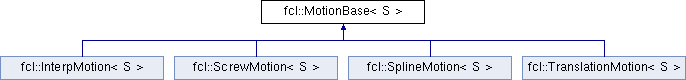FCL  0.6.0 Flexible Collision Library
fcl::MotionBase< S > Class Template Referenceabstract
Inheritance diagram for fcl::MotionBase< S >:## Public Member Functions

virtual bool integrate (S dt) const =0
Integrate the motion from 0 to dt.

virtual S computeMotionBound (const BVMotionBoundVisitor< S > &mb_visitor) const =0
Compute the motion bound for a bounding volume, given the closest direction n between two query objects.

virtual S computeMotionBound (const TriangleMotionBoundVisitor< S > &mb_visitor) const =0
Compute the motion bound for a triangle, given the closest direction n between two query objects.

void getCurrentTransform (Matrix3< S > &R, Vector3< S > &T) const
Get the rotation and translation in current step.

void getCurrentTransform (Quaternion< S > &Q, Vector3< S > &T) const

void getCurrentRotation (Matrix3< S > &R) const

void getCurrentRotation (Quaternion< S > &Q) const

void getCurrentTranslation (Vector3< S > &T) const

virtual void getCurrentTransform (Transform3< S > &tf) const =0

virtual void getTaylorModel (TMatrix3< S > &tm, TVector3< S > &tv) const =0

const std::shared_ptr< TimeInterval< S > > & getTimeInterval () const

## Protected Attributes

std::shared_ptr< TimeInterval< S > > time_interval_

The documentation for this class was generated from the following files: